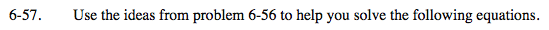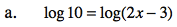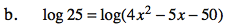### Home > INT3 > Chapter Ch6 > Lesson 6.2.3 > Problem6-57

6-57.
1. Use the ideas from problem 6‑56 to help you solve the following equations. Homework Help ✎

1. log(10) = log(2x – 3)

2. log(25) = log(4x2 – 5x – 50)If log a = log b, then a = b.

10 = 2x − 3

$x = 6.5$See part (a).